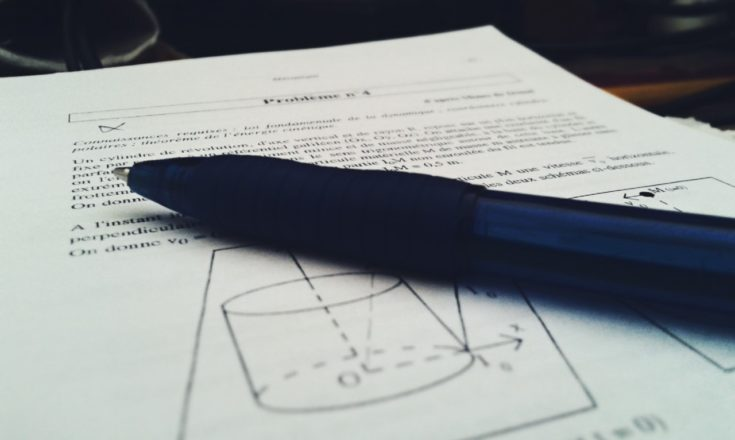# PHYSICS TEACHER

## Physics Fundamentals BLOG for K12RSS Feed

# cbse physics### Physics problems for class 9 – numericals with solutions

##### Force Gravitation Motion Newton's Law of Motion Question Set with Numerical Problems

Physics problems for class 9 (CBSE)–numericals set1 This set of Physics problems for class 9 students of CBSE/ICSE/HS boards cover Newton’s Laws of motion, Force, linear motion equations and Gravitation. Hope the students will like this set and try to solve the numerical problems themselves. I am providing the solutions as well, but you should